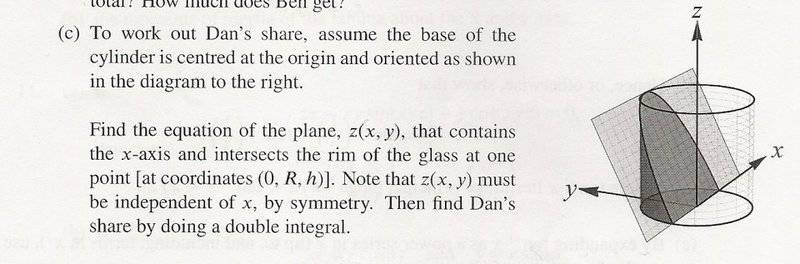# Double integral under a plane

## Homework Statement

Hi ! :) I'm having some difficulties with the question below, in which there are numerous steps and I am unsure in which part(/s!) I have gone wrong.

The question is as below; you must via integration calculate the shaded volume of a perfect cylinder of radius R and height h. The question wants you to do it as an integration under a plane I believe, so I have attempted to do so.## The Attempt at a Solution

Equation of a plane can be described by 3 points, which I have chosen as (0,R,h),(0,0,0) and (1,0,0). From this i have two vectors, A (0,-R,-h) and B(1,-R, -h) for which I have done the cross product to find the equation of a plane:
-hy +Rz= 0
I then integrate in polar coordinates over over the surface, which I believe is ∫∫z(x,y) dA = (h/R)∫∫r2sinθ dr dθ with the limits being 0≤ r ≤ R and 0 ≤ θ ≤π, which gives me the answer of 2R2h/3. I am dubious of this answer, as looking at the symmetry of the container I would assume it was (πR2/4)h.

Any clues towards where I went wrong would be highly appreciated!

Last edited:

BvU
Homework Helper
Hi,
Is Dan's share a volume fraction or an area ?

Hi! :)
A volume fraction of the cylinder, apologies; the shaded volume, not the shaded area!

LCKurtz
•